## If y varies directly as x, and y is 48 when x is 6, which expression can be used to find the value of y when x is 2? y = StartFraction 48 Ov

Question

If y varies directly as x, and y is 48 when x is 6, which expression can be used to find the value of y when x is 2? y = StartFraction 48 Over 6 EndFraction (2)

in progress 0
2 weeks 2021-09-05T14:25:54+00:00 1 Answers 0 views 0

The expression that can be used to find the value of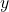when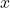is 2 is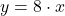(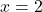)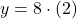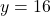Step-by-step explanation:

As we know thatvaries directly as. The following expression is done: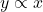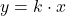Where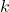is the proportionality constant.

If we know that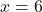and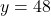, the proportionality constant is: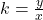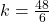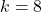The expression that can be used to find the value ofwhenis 2 is()